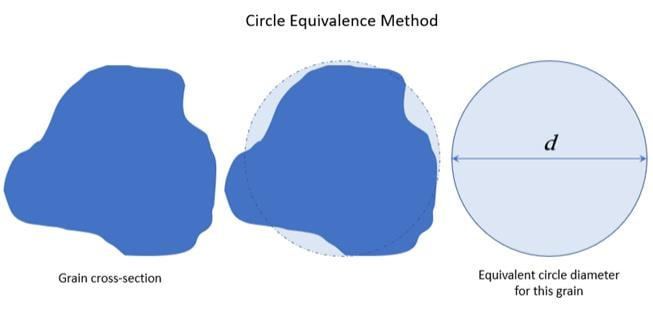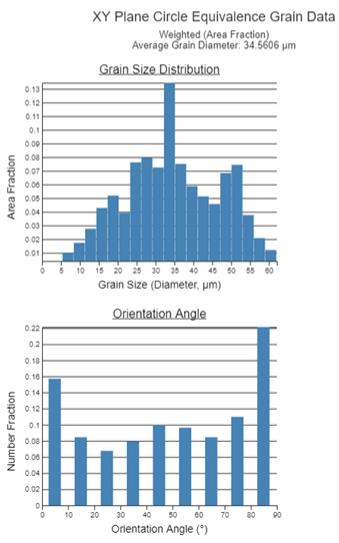## General Mechanical

•dingyangyao1995
Subscriber

Hello,

I was wondering how the Equivalent Circle Method is applied in the Additive Science Manual? Is the boundary of the grain calculated using some line integral to obtain the area and then use the area to calculate the diameter?

Thanks

•Sandeep Medikonda
Ansys Employee

Hi, The details of the equivalent circle method are discussed here (Pages 9-11).

For each grain, the area and the area fraction of the total area are calculated. To do this, the diameter of an equivalent circle for each grain is calculated using the Circle Equivalence Method. Grain area and diameter values for all the grains are then aggregated.•dingyangyao1995
Subscriber

I already read through this part about the documentation. However, my question is how the Circle Equivalence Method is used. What are the equations used to transform the non-uniform area into a circle? how did you estimate the diameter or the circle? using Greene's theorem?

I guess my question would be what is the circle equivalence method.

•yizhang1
Ansys Employee

It is not as complicated as Greene's theorem.

In the background, the microstructure is calculated based on a structured grid (like voxels, but way smaller than that). The program will evaluate the area, A, of each grain based on how many grid it covers. And then it will be easy to evaluate the equivalent circle diameter, D, for this grain: A=PI(D/2)^2.

The purpose is to represent the size of a grain by one singe value, D, other than length, height...